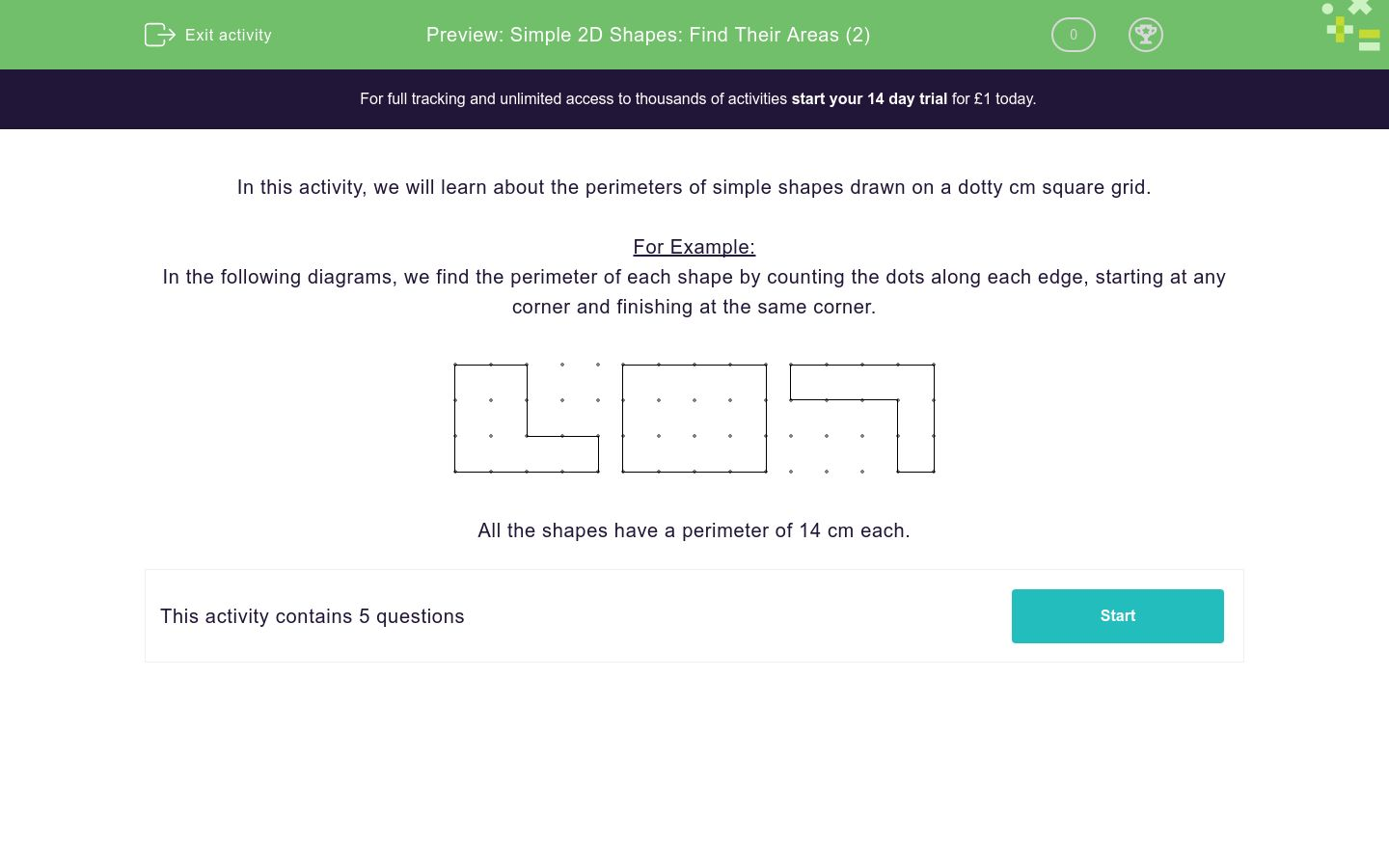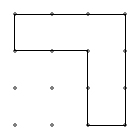# Simple 2D Shapes: Find Their Areas (2)

In this worksheet, students calculate the area of simple 2D shapes drawn on a dotty cm grid by counting along the edges.Key stage:  KS 2

Curriculum topic:   Measurement

Curriculum subtopic:   Calculate Areas

Difficulty level:### QUESTION 1 of 10

In this activity, we will learn about the perimeters of simple shapes drawn on a dotty cm square grid.

For Example:

In the following diagrams, we find the perimeter of each shape by counting the dots along each edge, starting at any corner and finishing at the same corner.All the shapes have a perimeter of 14 cm each.

Find the perimeter of this shape in cm.Just write the number.

Find the perimeter of this shape in cm.Just write the number.

Find the perimeter of this shape in cm.Just write the number.

Find the perimeter of this shape in cm.Just write the number.

Find the perimeter of this shape in cm.Just write the number.

• Question 1

Find the perimeter of this shape in cm.Just write the number.

14
EDDIE SAYS
Find the perimeter of the shape by counting the dots along each edge, starting in any corner. There are 14, so the perimeter is 14.
• Question 2

Find the perimeter of this shape in cm.Just write the number.

16
EDDIE SAYS
Find the perimeter of the shape by counting the dots along each edge, starting in any corner. There are 16, so the perimeter is 16.
• Question 3

Find the perimeter of this shape in cm.Just write the number.

14
EDDIE SAYS
Find the perimeter of the shape by counting the dots along each edge, starting in any corner. There are 14, so the perimeter is 14.
• Question 4

Find the perimeter of this shape in cm.Just write the number.

12
EDDIE SAYS
Find the perimeter of the shape by counting the dots along each edge, starting in any corner. There are 12, so the perimeter is 12.
• Question 5

Find the perimeter of this shape in cm.Just write the number.

14
EDDIE SAYS
Find the perimeter of the shape by counting the dots along each edge, starting in any corner. There are 14, so the perimeter is 14.
---- OR ----

Sign up for a £1 trial so you can track and measure your child's progress on this activity.

### What is EdPlace?

We're your National Curriculum aligned online education content provider helping each child succeed in English, maths and science from year 1 to GCSE. With an EdPlace account you’ll be able to track and measure progress, helping each child achieve their best. We build confidence and attainment by personalising each child’s learning at a level that suits them.

Get started